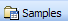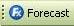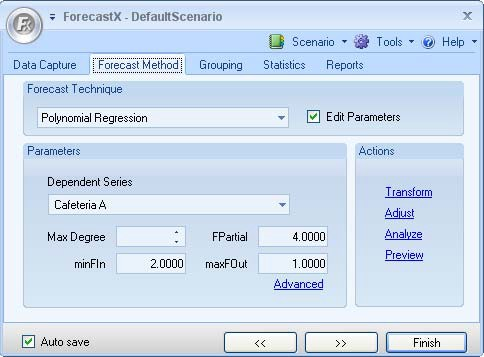# Polynomial Regression

Polynomial Regression models can contain one, two, or even several Independent Variables similar to that of a Multiple Regression model. However, Polynomial Regression goes further and treats the relationship between the Dependent and Independent Variable in more than a linear way. Polynomial Regression enables the Independent Variables to be present in various powers.

To use the Polynomial Regression forecasting technique:

1. Click onand open the ForecastingExamples.xls file.
Note: The ForecastingExamples.xls file is a data example to demonstrate how the Polynomial Regression method is used. For your company’s purposes, you will have your own data available.
2. Click on the Polynomial Regression sheet.
3. Click in a cell containing data and open ForecastX by clicking on. The Data Capture window will be displayed.
4. Click on the Forecast Method tab and select Polynomial Regression as your forecasting technique.5. In the Parameters area, configure the options available. The following table details what each option means.
 Parameter Description Dependent Series Select the dependent series of the Polynomial Regression. maxDegree Determine the maximum possible degree of Independent Variables in the model. It is recommended that no more than three is used. FPartial F-value used to determine the highest degree of the Independent Variables used in the model. minFin Determine the minimum F-value of an Independent Variable that is used in the model. Note: This is a Stepwise Regression parameter that can help determine the most significant Independent Variables in the model. maxFout Determine the maximum F-value of an Independent Variable that is used in the model. Note: This is a Stepwise Regression parameter that can help determine the most significant Independent Variables in the model.
6. On the Reports tab, select the Audit Trail report and ensure that all other reports are not selected.
7. Click Finish.